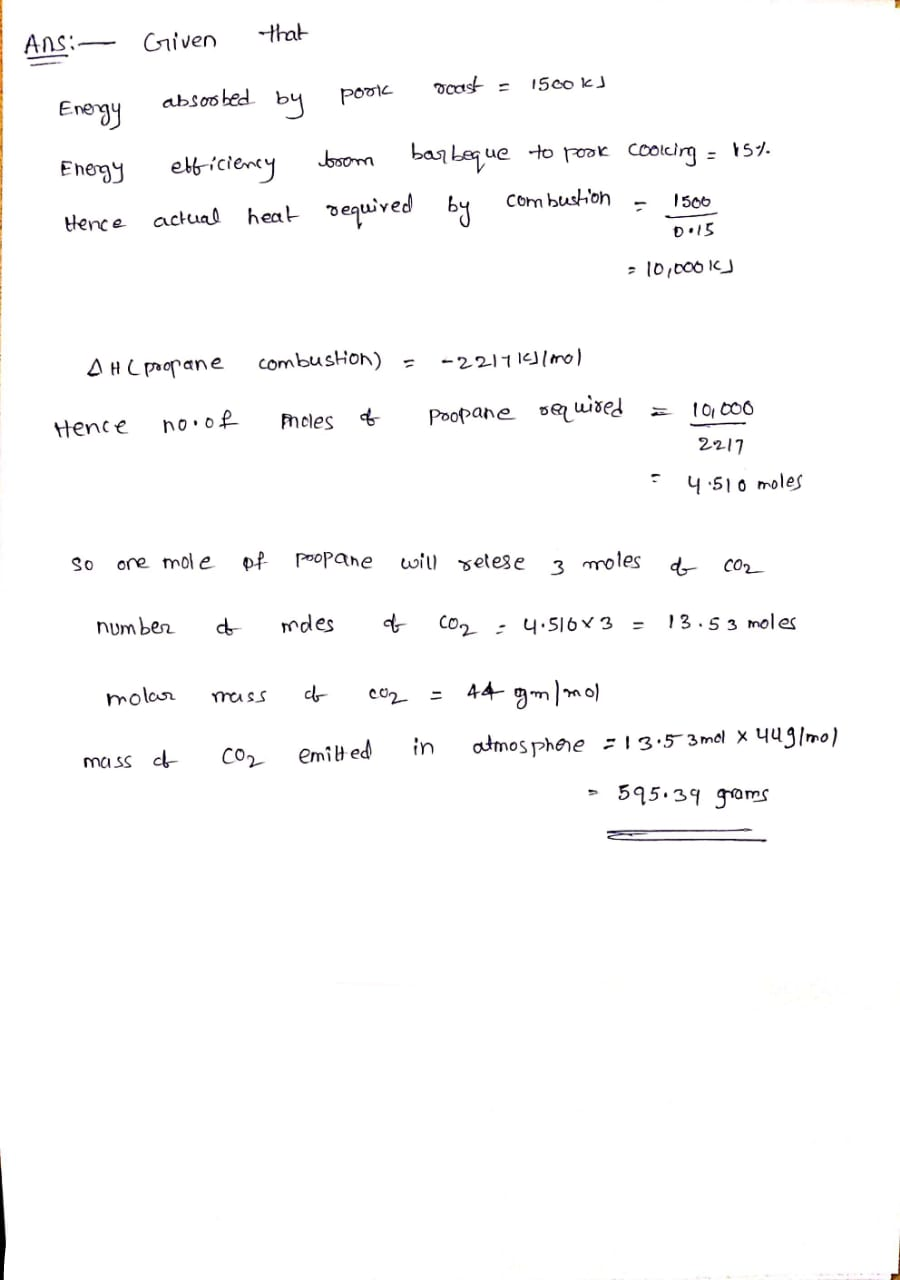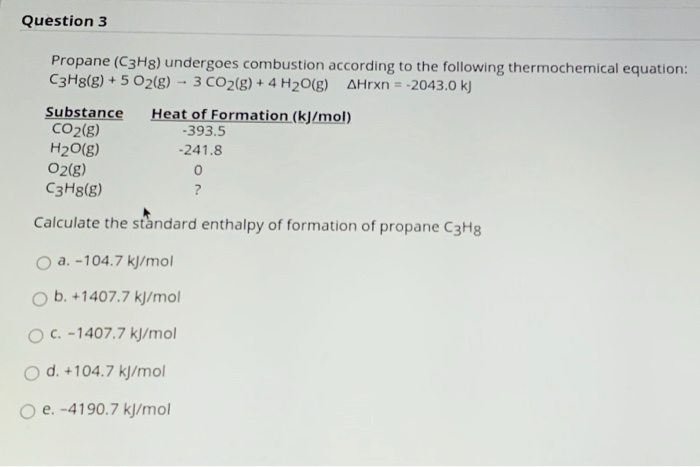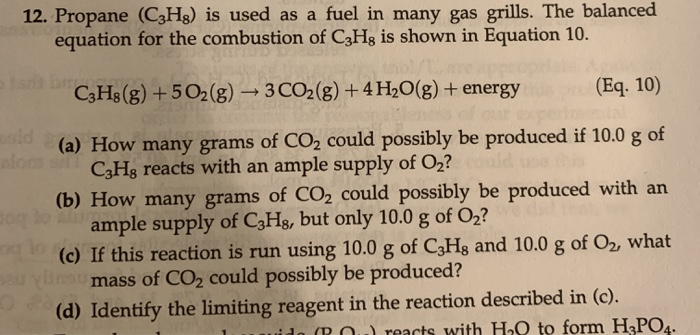Question

# The propane fuel (C3H8) used in gas barbeques burns according to this thermochemical equation. If a...

The propane fuel (C3H8) used in gas barbeques burns according to this thermochemical equation. If a pork roast must absorb 1.5×103 kJ to fully cook, and if only 15 % of the heat produced by the barbeque is actually absorbed by the roast, what mass of CO2 is emitted into the atmosphere during the grilling of the pork roast?#### Earn Coins

Coins can be redeemed for fabulous gifts.

Similar Homework Help Questions
• ### The propane fuel (C3H8) used in gas barbeques burns according to this thermochemical equation. C3H8(g)+5O2(g)→3CO2(g)+4H2O(g)ΔH∘rxn=−2044kJ If...

The propane fuel (C3H8) used in gas barbeques burns according to this thermochemical equation. C3H8(g)+5O2(g)→3CO2(g)+4H2O(g)ΔH∘rxn=−2044kJ If a pork roast must absorb 1.7×103 kJ to fully cook, and if only 15 % of the heat produced by the barbeque is actually absorbed by the roast, what mass of CO2 is emitted into the atmosphere during the grilling of the pork roast?

• ### The propane fuel (C3H8) used in gas barbeques burns according to the following thermochemical equation: C3H8(g)+5O2(g)...

The propane fuel (C3H8) used in gas barbeques burns according to the following thermochemical equation: C3H8(g)+5O2(g)?3CO2(g)+4H2O(g) If a pork roast must absorb 1700kJ to fully cook, and if only 14% of the heat produced by the barbeque is actually absorbed by the roast, what mass of CO2 is emitted into the atmosphere during the grilling of the pork roast? Express your answer using two significant figures.

• ### The propane fuel (C3H8) used in gas barbeques burns according to the following thermochemical equation: C3H8(g)+5O2(g)...

The propane fuel (C3H8) used in gas barbeques burns according to the following thermochemical equation:C3H8(g)+5O2(g)→3CO2(g)+4H2O(g) ΔH rxn= 2217kJPart AIf a pork roast must absorb 1500kJ to fully cook, and if only 12% of the heat produced by the barbeque is actually absorbed by the roast, what mass of CO2 is emitted into the atmosphere during the grilling of the pork roast?Express your answer using two significant figures. m=_____________________g

• ### he propane fuel (C3H8) used in gas BBQs burns according to this thermochemical equation: C3H8 +...

he propane fuel (C3H8) used in gas BBQs burns according to this thermochemical equation: C3H8 + 5O2 --> 3CO2 + 4H2O delta H = -2217kJ If a pork roast must absorb 1.6x10^3 kJ to fully cook, and if ONLY 10% of the heat produced by the BBQ is actually absorbed by the roast, what mass of CO2 is emitted into the atmosphere during the grilling of the pork roast.

• ### The propane fuel (C3H8) used in gas barbecues burns according to the following equation: C3H8(g)+5O2(g)→3CO2(g)+4H2O(g)ΔH∘rxn=−2044kJ PART...

The propane fuel (C3H8) used in gas barbecues burns according to the following equation: C3H8(g)+5O2(g)→3CO2(g)+4H2O(g)ΔH∘rxn=−2044kJ PART A: If a pork roast must absorb 2.3×103kJ to fully cook, and if only 10.% of the heat produced by the barbecue is actually absorbed by the roast, what mass of CO2 is emitted into the atmosphere during the grilling of the pork roast?

• ### If a pork roast must absorb 1500kJ to fully cook, and if only 11% of the...

If a pork roast must absorb 1500kJ to fully cook, and if only 11% of the heat produced by the barbeque is actually absorbed by the roast, what mass of CO2 is emitted into the atmosphere during the grilling of the pork roast? Express your answer using two significant figures. The propane fuel (C3H8) used in gas barbeques burns according to the following thermochemical equation: C3H8(g)+5O2(g)→3CO2(g)+4H2O(g)ΔH∘rxn=−2044kJ

• ### Question 3 Propane (C3H8) undergoes combustion according to the following thermochemical equation: C3H8(g) + 5 O2(g)...Question 3 Propane (C3H8) undergoes combustion according to the following thermochemical equation: C3H8(g) + 5 O2(g) -- 3 CO2(g) + 4H2O(g) Arxn = -2043.0 kJ Substance Heat of Formation (kJ/mol) CO2(g) -393.5 H2O(g) -241.8 O2(g) 0 C3H8(g) ? Calculate the standard enthalpy of formation of propane C3H8 a. -104.7 kJ/mol ob. +1407.7 kJ/mol C. -1407.7 kJ/mol O d. +104.7 kJ/mol o e. -4190.7 kJ/mol

• ### The combustion of propane gas (C3H8) is used to fuel barbeque grills. In order to produce...

The combustion of propane gas (C3H8) is used to fuel barbeque grills. In order to produce 5.65 moles of water how many moles of oxygen gas are needed? C3H8(g) + 5 O2(g) → 3 CO2(g) + 4 H2O(l

• ### 8.24 kg of propane gas is being used as fuel. Based on the reaction C3H8 +...

8.24 kg of propane gas is being used as fuel. Based on the reaction C3H8 + O2 ? CO2 + H2O, how many kilograms of carbon dioxide have been produced? (Note: Please make sure to balance the equation) 2.75 kg 8.24 kg 24.72 kg 31.26 kg

• ### 12. Propane (C3H8) is used as a fuel in many gas grills. The balanced equation for the combustion of C3Hg is shown...12. Propane (C3H8) is used as a fuel in many gas grills. The balanced equation for the combustion of C3Hg is shown in Equation 10. C3H8(g) +502(g) + 3 CO2(g) + 4H2O(g) + energy (Eq. 10) (a) How many grams of CO2 could possibly be produced if 10.0 g of CzHg reacts with an ample supply of Oz? (b) How many grams of CO2 could possibly be produced with an ample supply of C3Hg, but only 10.0 g of O2?...